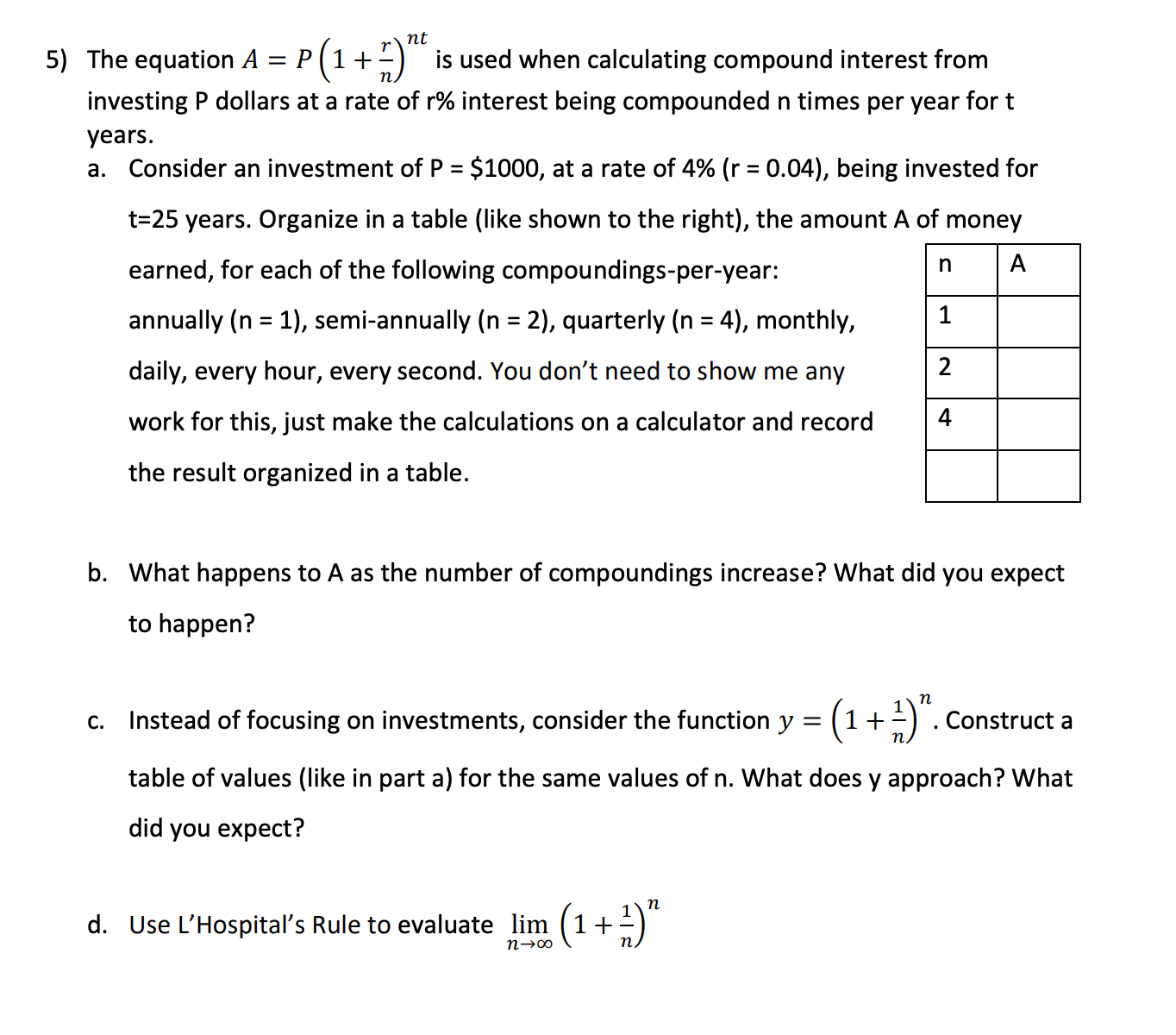# nt The equation A = P + is used when calculating compound interest from investing P dollars at a rate of r% interest being compounded n times per year for t years. a. Consider an investment ofP = $1000, at a rate of 4% (r = 0.04), being invested for %3D t=25 years. Organize in a table (like shown to the right), the amount A of money n A earned, for each of the following compoundings-per-year: annually (n = 1), semi-annually (n = 2), quarterly (n = 4), monthly, %3D %3D daily, every hour, every second. You don't need to show me any 2 work for this, just make the calculations on a calculator and record the result organized in a table. b. What happens to A as the number of compoundings increase? What did you expect to happen? c. Instead of focusing on investments, consider the function y = (1+±) . Construct a table of values (like in part a) for the same values of n. What does y approach? What did you expect? п d. Use L'Hospital's Rule to evaluate lim (1+ n→∞ Questionhelp_outlineImage Transcriptionclosent The equation A = P + is used when calculating compound interest from investing P dollars at a rate of r% interest being compounded n times per year for t years. a. Consider an investment ofP =$1000, at a rate of 4% (r = 0.04), being invested for %3D t=25 years. Organize in a table (like shown to the right), the amount A of money n A earned, for each of the following compoundings-per-year: annually (n = 1), semi-annually (n = 2), quarterly (n = 4), monthly, %3D %3D daily, every hour, every second. You don't need to show me any 2 work for this, just make the calculations on a calculator and record the result organized in a table. b. What happens to A as the number of compoundings increase? What did you expect to happen? c. Instead of focusing on investments, consider the function y = (1+±) . Construct a table of values (like in part a) for the same values of n. What does y approach? What did you expect? п d. Use L'Hospital's Rule to evaluate lim (1+ n→∞ fullscreen

### Want to see this answer and more?

Experts are waiting 24/7 to provide step-by-step solutions in as fast as 30 minutes!*

*Response times may vary by subject and question complexity. Median response time is 34 minutes for paid subscribers and may be longer for promotional offers.
Tagged in
MathCalculus

### Other